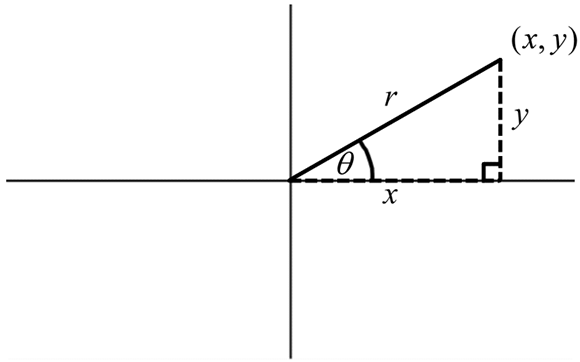Chapter 14

Graphing Polar Equations

In This ChapterChanging the mode and window of your calculatorEntering and graphing polar equationsUsing Trace to evaluate polar equationsViewing the table of a polar graphFinding the derivative of polar equations

A polar coordinate system is used in Pre-calculus class as yet another way to define a point. Polar coordinates are of the form (r, θ). The distance from the pole (similar to the origin) is called, r. The polar axis is a ray that extends from the pole (similar to the positive x-axis). A positive angle is measured in a counterclockwise direction from the polar axis to a line that connects the pole and a point. See Figure 14-1 for a visual of a polar coordinate.Figure 14-1: Polar coordinates.

Polar coordinates (r, θ) can be converted to rectangular coordinates (x, y), as discussed in Chapter 9. The purpose of this chapter is to explain ...

Get Ti-84 Plus Graphing Calculator For Dummies, 2nd Edition now with the O’Reilly learning platform.

O’Reilly members experience live online training, plus books, videos, and digital content from nearly 200 publishers.